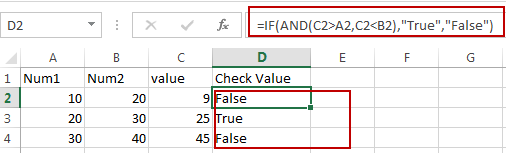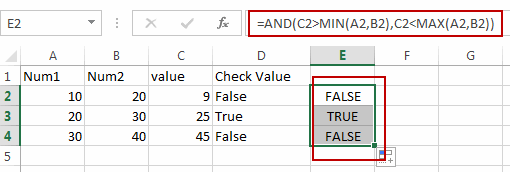# How to Check If value Is between Two Numbers in Excel

This post will guide you how to check if a cell value is between two numbers in Excel. How do I build an IF statement that test if a cell value is between two given values in formula in Excel.

## Check Value If It Is between Two Numbers

Assuming that you have list of data in range A1:C4, which contain numbers. And you need to test if a numeric value in Cell C2 is between two numbers in Range: A2:B2. How to do it. You can use IF function and the AND function to build an IF statement to check values. Like this:

`=IF(AND(C2>A2,C2<B2),"True","False")`

Type this formula into a blank cell which you want to place the result, and press Enter key on your keyboard. Then drag the AutoFill Handle down to cell C4 to apply this formula.Note: this formula is only valid when the Cell value in A2 is smaller than the cell value in B2.

You can also use another formula based on the AND function, the MIN function and the MAX function to achieve the same result. Like this:

`=AND(C2>MIN(A2,B2),C2<MAX(A2,B2))`### Related Functions

• Excel MIN function
The Excel MIN function returns the smallest numeric value from the numbers that you provided. Or returns the smallest value in the array.The MIN function is a build-in function in Microsoft Excel and it is categorized as a Statistical Function.The syntax of the MIN function is as below:= MIN(num1,[num2,…numn])….
• Excel MAX function
The Excel MAX function returns the largest numeric value from the numbers that you provided. Or returns the largest value in the array.= MAX(num1,[num2,…numn])…
• Excel IF function
The Excel IF function perform a logical test to return one value if the condition is TRUE and return another value if the condition is FALSE. The IF function is a build-in function in Microsoft Excel and it is categorized as a Logical Function.The syntax of the IF function is as below:= IF (condition, [true_value], [false_value])….
• Excel AND function
The Excel AND function returns TRUE if all of arguments are TRUE, and it returns FALSE if any of arguments are FALSE.The syntax of the AND function is as below:= AND (condition1,[condition2],…)…

Sidebar